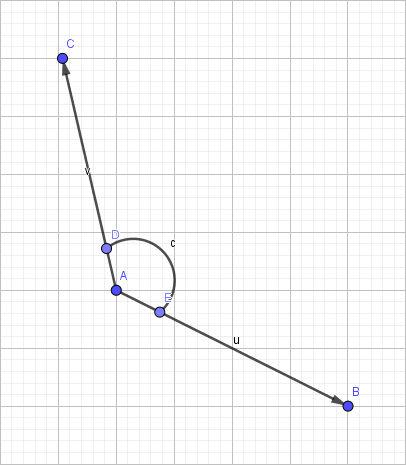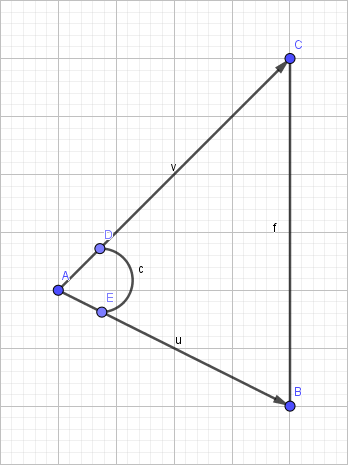# BZOJ1033：[ZJOI2008]杀蚂蚁

①如果地图上没有六只蚂蚁并且$$(0,0)$$没有蚂蚁就生产一只新蚂蚁。

②所以蚂蚁按照出生顺序开始依次移动。

③炮塔开始攻击。$$f$$为激光，$$C、B$$一个是炮塔一个是$$target$$$$A$$是蚂蚁，角$$c$$是锐角，那么$$A$$$$f$$的距离就是过$$A$$点作$$f$$的垂线的长度。那么可以用$$abs(v\times u)/|f|$$来表示，因为$$abs(v\times u)/2$$就是三角形$$ABC$$的面积（不懂的同学自己去看叉积），然后判断是否大于$$0.5$$就可以了。

④判断蚂蚁是否死亡，判断游戏是否结束。

#include <cmath>
#include <cstdio>
#include <iostream>
#include <algorithm>
using namespace std;
#define sqr(a) ((a)*(a))

const int inf=2e9;

double HP=1;//存1.1^k
int phe;//存每个点的信息素
int dx={0,1,0,-1};
int dy={1,0,-1,0};//从0到3分别是向东、西、南、北
int n,m,s,d,r,T,ant_cnt,ant_tot;
//n,m是地图大小，s是炮塔总个数，d是炮塔A一下的伤害，r是炮塔攻击范围，T是时间；
//ant_cnt动态储存场上蚂蚁的信息，ant_tot存蚂蚁总数，用于计算蚂蚁等级
bool obs,cake_in_map=1,game_over;
//obs[i][j]储存(i,j)是否有障碍物（蚂蚁或者炮塔），cake_in_map表示蛋糕是否在(n,m),game_over如字面意思。

int x=0,f=1;char ch=getchar();
for(;ch<'0'||ch>'9';ch=getchar())if(ch=='-')f=-1;
for(;ch>='0'&&ch<='9';ch=getchar())x=(x<<1)+(x<<3)+ch-'0';
return x*f;
}//快读

struct Map {
int x,y,id;//x,y表示坐标,id表示id号方向

Map(){};
Map(int _x,int _y,int _id) {
x=_x,y=_y,id=_id;
}

bool operator <(const Map &a)const {
if(phe[x][y]==phe[a.x][a.y])return id<a.id;
return phe[x][y]>phe[a.x][a.y];
}//按照phe为第一关键字，id为第二关键字排序
};//存可达点信息

struct Ant {
int x,y,birth,lv,hp,cake,hp_max,lstx,lsty;
//x,y存蚂蚁坐标，birth是出生时间，lv是等级，hp是当前生命值，hp_max是最大生命值
//cake表示是否背上了蛋糕，lstx,lsty表示该蚂蚁上一秒在哪个点
bool operator <(const Ant &a)const {
return birth<a.birth;
}//按出生时间排序

void clear() {
birth=inf;
lstx=lsty=x=y=lv=hp=cake=hp_max=0;
}//初始化

bool check(int X,int Y) {
return X<0||X>n||Y<0||Y>m||obs[X][Y];//(X,Y)是否可以到达
}

void new_born(int tim) {
ant_cnt++;ant_tot++;//场上蚂蚁数+1，该蚂蚁编号为ant_tot;
obs=1;lstx=lsty=x=y=0;//初始化
if((ant_tot-1)%6==0)HP=1.1*HP;//每过6只蚂蚁升一级
hp_max=hp=(int)(HP*4);
birth=tim;cake=0;
lv=(ant_tot-1)/6+1;//初始化新生蚂蚁信息
}

int calc(int a,int b) {
if(a<b)return 4-(b-a);
return a-b;
}//算从a号方向逆时针旋转几次可以到b号方向

void move(int tim,bool way) {
if(cake)phe[x][y]+=5;
else phe[x][y]+=2;//先在原格子上留下信息素
Map a;int top=-1;
for(int i=0;i<4;i++) {
int X=x+dx[i],Y=y+dy[i];
if(check(X,Y))continue;//如果该点为不可达点
if(X==lstx&&Y==lsty)continue;//或者该点为上一秒所在的点
a[++top]=Map(X,Y,i);//该点可达
}if(top==-1){//如果被卡在原地了
lstx=x,lsty=y;//一定要记得更新lstx,lsty
if(x==n&&y==m&&cake_in_map) {//一定要记得更新是否拿起了蛋糕
cake_in_map=0;
cake=1;
hp=min(hp+hp_max/2,hp_max);
}
return;
}
obs[x][y]=0;//移动的时候记得更新obs数组
sort(a,a+top+1);//按phe为第一关键字，id为第二关键字排序
int goal=0;//0号点就是目标点
if(way) {//如果活动时间是5的倍数
swap(a,a[goal]);goal=-1;//首先把goal放到0来，然后从剩下的几个点里找可以逆时针到达的
for(int i=1;i<=top;i++)
if(goal==-1)goal=i;//因为goal初始值是-1，所以必须这么写，不然会RE
else {
if(calc(a.id,a[goal].id)>calc(a.id,a[i].id))
goal=i;//如果从0开始逆时针旋转到i相对于goal用了更少的次数那么i肯定更优
}
if(goal==-1)goal=0;//如果不存在了，说明转了一圈，就回到了原本的goal
}
lstx=x,lsty=y;
x=a[goal].x,y=a[goal].y;//移动
if(x==n&&y==m&&cake_in_map) {
cake_in_map=0;
cake=1;
hp=min(hp+hp_max/2,hp_max);
}//判断是否背起了蛋糕
obs[x][y]=1;//新到达点成为障碍点
}

void check_life() {
if(hp>=0&&cake&&(!x)&&(!y)) {
game_over=1;return;
}//如果该蚂蚁活着把蛋糕送到了蚂蚁窝那么游戏结束
if(hp>=0)return;//如果他是活着的蚂蚁就不管他
birth=inf;//birth改成inf
obs[x][y]=0;//清楚障碍记录
if(cake)cake_in_map=1;//如果背着蛋糕那么蛋糕归为
ant_cnt--;//场上蚂蚁数-1
}
}ant;

struct Turret {
int x,y;//炮塔坐标

double dis(int x1,int y1,int x2,int y2) {
return sqrt(sqr(x1-x2)+sqr(y1-y2));
}//求(x1,y1)(x2,y2)两点之间的距离

int dot_pro(int x1,int y1,int x2,int y2) {
return x1*x2+y1*y2;
}//求向量(x1,y1)(x2,y2)的点积

int cross_pro(int x1,int y1,int x2,int y2) {
return abs(x1*y2-y1*x2);
}//求向量(x1,y1)(x2,y2)的叉积

bool check_attack(int x1,int y1,int x2,int y2,int X,int Y) {
if(dot_pro(X-x1,Y-y1,x2-x1,y2-y1)<0)
return dis(x1,y1,X,Y)<=0.5;//如果点积小于0说明该角是钝角
if(dot_pro(X-x2,Y-y2,x1-x2,y1-y2)<0)
return dis(X,Y,x2,y2)<=0.5;//同上
return 1.0*cross_pro(x1-X,y1-Y,x2-X,y2-Y)/dis(x1,y1,x2,y2)<=0.5;//否则直接求距离
}

void Attack() {
int goal=0;double Dis=inf;//goal存目标蚂蚁编号，Dis存该蚂蚁离炮塔的距离
for(int i=1;i<=ant_cnt;i++) {
double tmp=dis(ant[i].x,ant[i].y,x,y);//i号蚂蚁与当前炮塔距离
if(tmp>r)continue;//距离大于攻击范围直接pass
if(ant[i].cake) {
goal=i;
break;
}//如果该蚂蚁背着蛋糕并且在攻击范围内直接成为target
if(tmp<Dis) {
Dis=tmp;
goal=i;
}//否则就选最近的蚂蚁攻击
}
if(!goal)return;//如果打不到蚂蚁就return
int X=ant[goal].x,Y=ant[goal].y;//存下target的坐标，表示激光的线段就是(x,y)——>(X,Y)
for(int i=1;i<=ant_cnt;i++) {//枚举蚂蚁
if(check_attack(x,y,X,Y,ant[i].x,ant[i].y))
//如果以(ant[i].x,ant[i].y)为圆心，半径为0.5的圆和线段(x,y)——>(X,Y)有交点
ant[i].hp-=d;//受到一次伤害
}
}
}tur;

void clear_phe() {
for(int i=0;i<=n;i++)
for(int j=0;j<=m;j++)
if(phe[i][j])phe[i][j]--;
}//信息素流失

int main() {
for(int i=1;i<=s;i++) {
obs[tur[i].x][tur[i].y]=1;
}//读入炮塔信息
for(int i=1;i<=6;i++)ant[i].clear();//先初始化，我用在inf秒出生表示该蚂蚁不存在或者挂掉了。
for(tim=1;tim<=T;tim++) {
sort(ant+1,ant+7);//先按出生时间排序
if(ant_cnt<6&&(!obs))
ant[ant_cnt+1].new_born(tim);//如果不够6只并且(0,0)没有蚂蚁就生产新蚂蚁
for(int i=1;i<=ant_cnt;i++)
ant[i].move(tim,(tim-ant[i].birth+1)%5==0);//移动
for(int i=1;i<=s;i++)tur[i].Attack();//炮塔一起攻击
for(int i=1;i<=ant_cnt;i++)ant[i].check_life();//判断蚂蚁是否死亡，游戏是否结束
if(game_over)break;//游戏提前结束
clear_phe();//信息素流失
}
if(tim<=T)printf("Game over after %d seconds\n",tim),tim--;//如果游戏是提前结束的那么最后一秒就不算数
else puts("The game is going on"),tim=T;//否则T就是结束时间
printf("%d\n",ant_cnt);
sort(ant+1,ant+7);
for(int i=1;i<=ant_cnt;i++)
printf("%d %d %d %d %d\n",tim-ant[i].birth+1,ant[i].lv,ant[i].hp,ant[i].x,ant[i].y);
return 0;
}


posted @ 2018-06-02 21:16  AKMer  阅读(294)  评论(4编辑  收藏  举报